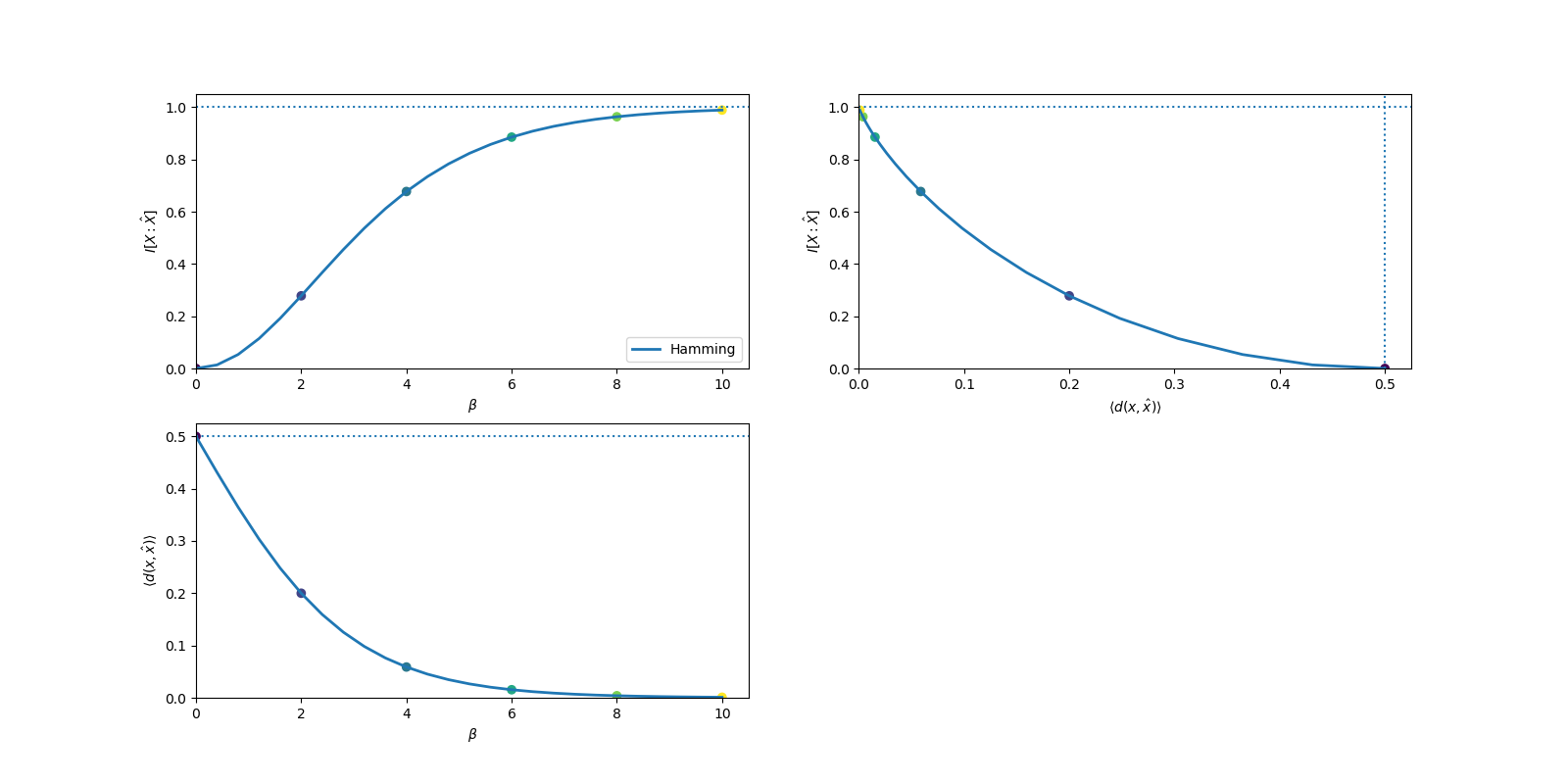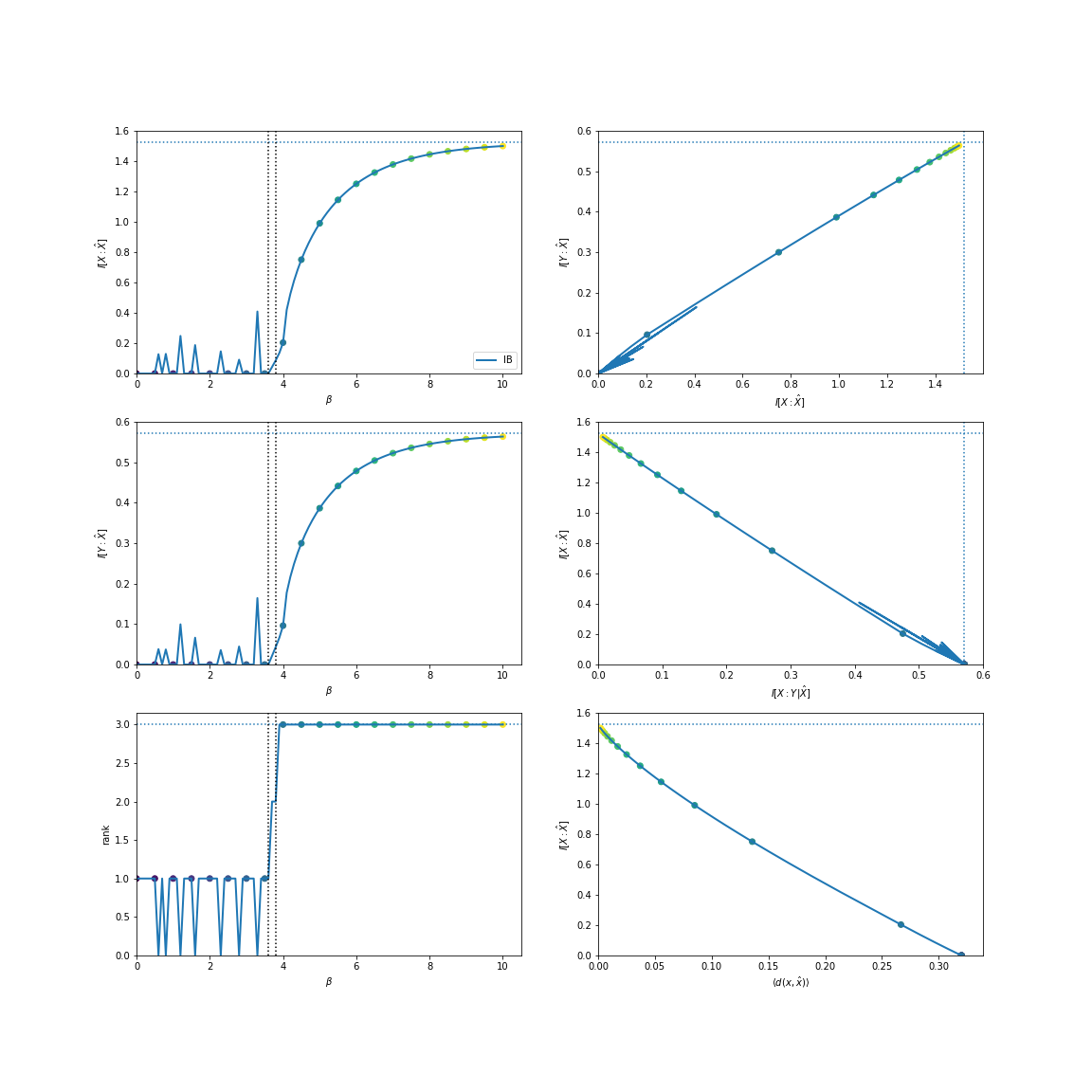# Rate Distortion Theory¶

Note

We use $$p$$ to denote fixed probability distributions, and $$q$$ to denote probability distributions that are optimized.

Rate-distortion theory [CT06] is a framework for studying optimal lossy compression. Given a distribution $$p(x)$$, we wish to find $$q(\hat{x}|x)$$ which compresses $$X$$ as much as possible while limiting the amount of user-defined distortion, $$d(x, \hat{x})$$. The minimum rate (effectively, code book size) at which $$X$$ can be compressed while maintaining a fixed distortion is known as the rate-distortion curve:

$R(D) = \min_{q(\hat{x}|x), \langle d(x, \hat{x}) \rangle = D} \I{X : \hat{X}}$

By introducing a Lagrange multiplier, we can transform this constrained optimization into an unconstrained one:

$\mathcal{L} = \I{X : \hat{X}} + \beta \langle d(x, \hat{x}) \rangle$

where minimizing at each $$\beta$$ produces a point on the curve.

## Example¶

It is known that under the Hamming distortion ($$d(x, \hat{x}) = \left[ x \neq \hat{x} \right]$$) the rate-distortion function for a biased coin has the following solution: $$R(D) = \H{p} - \H{D}$$:

In : from dit.rate_distortion import RDCurve

In : d = dit.Distribution(['0', '1'], [1/2, 1/2])

In : RDCurve(d, beta_num=26).plot();# Information Bottleneck¶

The information bottleneck [TPB00] is a form of rate-distortion where the distortion measure is given by:

$d(x, \hat{x}) = D\left[~p(Y | x)~\mid\mid~q(Y | \hat{x})~\right]$

where $$D$$ is an arbitrary divergence measure, and $$\hat{X} - X - Y$$ form a Markov chain. Traditionally, $$D$$ is the Kullback-Leibler Divergence, in which case the average distortion takes a particular form:

$\begin{split}\langle d(x, \hat{x}) \rangle &= \sum_{x, \hat{x}} q(x, \hat{x}) \DKL{ p(Y | x) || q(Y | \hat{x}) } \\ &= \sum_{x, \hat{x}} q(x, \hat{x}) \sum_{y} p(y | x) \log_2 \frac{p(y | x)}{q(y | \hat{x})} \\ &= \sum_{x, \hat{x}, y} q(x, \hat{x}, y) \log_2 \frac{p(y | x) p(x) p(y) q(\hat{x})}{q(y | \hat{x}) p(x) p(y) q(\hat{x})} \\ &= \sum_{x, \hat{x}, y} q(x, \hat{x}, y) \log_2 \frac{p(y | x) p(x)}{p(x) p(y)} \frac{p(y)q(\hat{x})}{q(y | \hat{x}) q(\hat{x})} \\ &= \I{X : Y} - \I{\hat{X} : Y}\end{split}$

Since $$\I{X : Y}$$ is constant over $$q(\hat{x} | x)$$, it can be removed from the optimization. Furthermore,

$\begin{split}\I{X : Y} - \I{\hat{X} : Y} &= (\I{X : Y | \hat{X}} + \I{X : Y : \hat{X}}) - (\I{Y : \hat{X} | X} + \I{X : Y : \hat{X}}) \\ &= \I{X : Y | \hat{X}} - \I{Y : \hat{X} | X} \\ &= \I{X : Y | \hat{X}}\end{split}$

where the final equality is due to the Markov chain. Due to all this, Information Bottleneck utilizes a “relevance” term, $$\I{\hat{X} : Y}$$, which replaces the average distortion in the Lagrangian:

$\mathcal{L} = \I{X : \hat{X}} - \beta \I{\hat{X} : Y} ~.$

Though $$\I{X : Y | \hat{X}}$$ is the most simplified form of the average distortion, it is faster to compute $$\I{\hat{X} : Y}$$ during optimization.

## Example¶

Consider this distribution:

In : d = dit.Distribution(['00', '02', '12', '21', '22'], [1/5]*5)


There are effectively three features that the fist index, $$X$$, has regarding the second index, $$Y$$. We can find them using the standard information bottleneck:

In : from dit.rate_distortion import IBCurve

In : IBCurve(d, beta_num=26).plot();We can also find them utilizing the total variation:

In : from dit.divergences.pmf import variational_distance

In : IBCurve(d, divergence=variational_distance).plot();Note

The spiky behavior at low $$\beta$$ values is due to numerical imprecision.

# APIs¶

class RDCurve(dist, rv=None, crvs=None, beta_min=0, beta_max=10, beta_num=101, alpha=1.0, distortion=Distortion(name='Hamming', matrix=<function hamming_distortion>, optimizer=<class 'dit.rate_distortion.rate_distortion.RateDistortionHamming'>), method=None)[source]

Compute a rate-distortion curve.

class IBCurve(dist, rvs=None, crvs=None, rv_mode=None, beta_min=0.0, beta_max=15.0, beta_num=101, alpha=1.0, method='sp', divergence=None)[source]

Compute an information bottleneck curve.